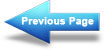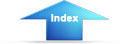Custom SearchDesign: Solar Heating of Buildings and Domestic Hot WaterMIL-HDBK-1003/13A
Current NAVFAC instructions should be consulted to see which factors apply to
which projects.  Tables are supplied to allow each method to be calculated as
follows:
10% discount factors - Table 3-5a
0% discount factors - Table 3-5b
7% discount factors - Table 3-5c
Various differential escalation rates are given with each table.  Consult
NAVFAC P-442 for rates not given.  The tables are given for 25 years
lifetime.  Shorter periods can be calculated also.
The Savings Investment Ratio (SIR) compares the lifetime discounted savings
and costs with the initial costs as follows:
Initial cost of system
A ratio greater than 1.0 is desired indicating the project is cost effective.
Methods to calculate SIR either using a discount factor or not using one are
given in the next two sections.
3.7.3.1 Present worth analysis using a discount factor - Worksheet E-1 or
E-2.  The present worth of the lifetime energy savings is:
Fuel
savings
x (EFC) x (PWF)
3.7.1
Where:
a.
Fuel savings are calculated in Section 3.7.1.
b.
(EFC) - Escalates fuel cost from present cost to anticipated first
year occupancy.  See Table, Section 3.7.1.  Enter in Worksheet E-1,
column (5).
c.
(PWF) is present worth factor from Table 3-5a (10% discount) or
Table 3-5b (7% discount).
A year by year summary can be calculated on Worksheet E-2 or the answer may
be obtained by a single multiplication using factors from the tables.  For
example, the present worth of 25 years fuel saved with differential inflation
rates and discount factors are as follows:
For 10% discount, from Table 3-5a
Present worth
=
18.049 x value of fuel saved/yr - 7% inflation
=
20.050 x value of fuel saved/yr - 8% inflation
=
25.000 x value of fuel saved/yr - 10% inflation
110Integrated Publishing, Inc. - A (SDVOSB) Service Disabled Veteran Owned Small Business